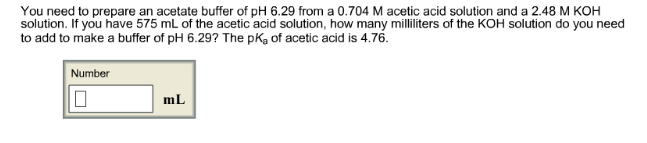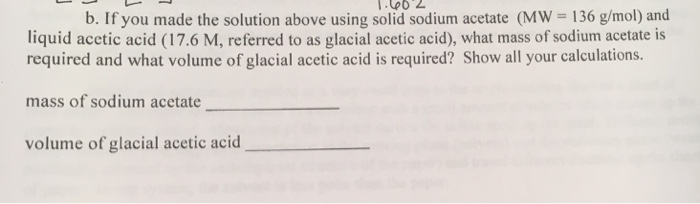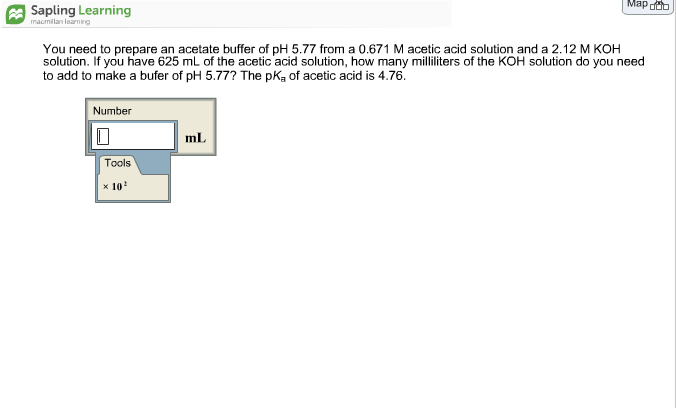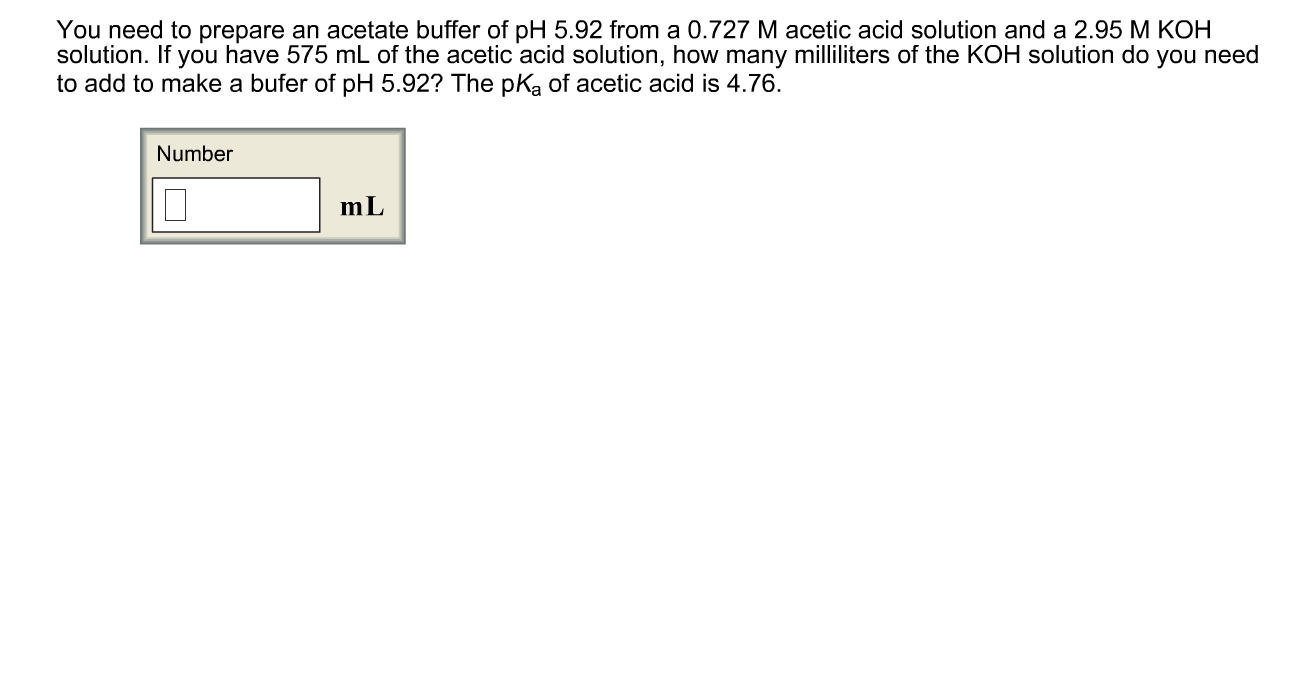Question

# Given an acetate buffer with pH 4.9, do you expect the solution to contain predominantly more...

Given an acetate buffer with pH 4.9, do you expect the solution to contain predominantly more undissociated acetic acid or more dissociated acetate ions? explain.

Henderson - Hasselbach equation is

pH = pKa + log([A-]/[HA])

where,

A- = conjucate base , CH3COO-

HA = weak acid , CH3COOH

pKa of CH3COOH = 4.75

4.9 = 4.75 + log([CH3COO-]/[CH3COOH])

log([CH3COO-] / [CH3COOH]) = 0.15

[CH3COO-] / [CH3COOH] = 1.413

Therefore,

The solution should contain predominentally more dissociated acetate ions.

#### Earn Coins

Coins can be redeemed for fabulous gifts.

Similar Homework Help Questions
• ### What reaction that occurs when HCl is added to a buffer solution composed of HC2H3O2 and...

What reaction that occurs when HCl is added to a buffer solution composed of HC2H3O2 and NaC2H3O2. What reaction that occurs when  is added to a buffer solution composed of  and . As HCl (H− and Cl+) is added, the H− will pair with C2H3O−2, neutralizing the acetate ion and becoming acetic acid. As HCl (H+ and Cl−) is added, the H+ will pair with HC2H3O2, neutralizing the acetate ion and becoming an acetic base., As HCl (H+ and Cl−) is added,...

• ### You want to make 500 mL of 0.400 M sodium acetate buffer pH 4.9. You have...

You want to make 500 mL of 0.400 M sodium acetate buffer pH 4.9. You have 17.5 M acetic acid and sodium acetate. Add 5.55 g of sodium acetate. How much acetic acid to add?

• ### You need to prepare an acetate buffer of pH 6.29 from a 0.704 M acetic acid...You need to prepare an acetate buffer of pH 6.29 from a 0.704 M acetic acid solution and a 2.48 M KOH solution. If you have 575 mL of the acetic acid solution, how many milliliters of the KOH solution do you need to add to make a buffer of pH 6.29? The pKa of acetic acid is 4.76. You need to prepare an acetate buffer of pH 6.29 from a 0.704 M acetic acid solution and a 2.48 M...

• ### You need to prepare 900 mL of a 0.25 M acetate buffer solution with a pH...

You need to prepare 900 mL of a 0.25 M acetate buffer solution with a pH of 4.3 a. Determine the concentration of both the acetate (conjugate base) and acetic acid (weak acid) in this solution. b. If you made the solution above using solid sodium acetate and liquid acetic acid, what mass of sodium acetate is required and what volume of glacial acetic acid is required?

• ### You need to prepare an acetate buffer of pH of 6.39 from a 0.876 M acetic...

You need to prepare an acetate buffer of pH of 6.39 from a 0.876 M acetic acid solution and a 2.52 M KOH solution. If you have 775mL of the acetic acid solution, how many milliliters of the KOH solution do you need to add to make a buffer of pH 6.39? The pKa of acetic acid is 4.76.

• ### You have prepared 250mL of a 0.200M acetate buffer solution with a pH of 4.53. b....You have prepared 250mL of a 0.200M acetate buffer solution with a pH of 4.53. b. If you made the solution above using solid sodium acetate (MW 136 g/mol) and liquid acetic acid (17.6 M, referred to as glacial acetic acid), what mass of sodium acetate is required and what volume of glacial acetic acid is required? Show all your calculations. mass of sodium acetate volume of glacial acetic acid

• ### You need to prepare an acetate buffer of pH 5.21 from a 0.635 M acetic acid...

You need to prepare an acetate buffer of pH 5.21 from a 0.635 M acetic acid solution and a 2.86 M KOH solution. If you have 830 mL of the acetic acid solution, how many milliliters of the KOH solution do you need to add to make a buffer of pH 5.21? The p?a of acetic acid is 4.76.

• ### You need to prepare an acetate buffer of pH 5.77 from a 0.671 M acetic acid...You need to prepare an acetate buffer of pH 5.77 from a 0.671 M acetic acid solution and a 2.12 M KOH solution. If you have 625 mL of the acetic acid solution, how many milliliters of the KOH solution do you need to add to make a buffer of pH 5.77? The pK_a of acetic acid is 4.76.

• ### You need to prepare an acetate buffer of pH 5.92 from a 0.727 M acetic acid...You need to prepare an acetate buffer of pH 5.92 from a 0.727 M acetic acid solution and a 2.95 M KOH solution. If you have 575 ml_of the acetic acid solution, how many milliliters of the KOH solution do you need to add to make a buffer of pH 5.92? The pKa of acetic acid is 4.76.

• ### You need to prepare an acetate buffer of pH 6.31 from a 0.848 M acetic acid...

You need to prepare an acetate buffer of pH 6.31 from a 0.848 M acetic acid solution and a 2.32 M KOH solution. If you have 675 mL of the acetic acid solution, how many milliliters of the KOH solution do you need to add to make a buffer of pH 6.31? The pKa of acetic acid is 4.76.